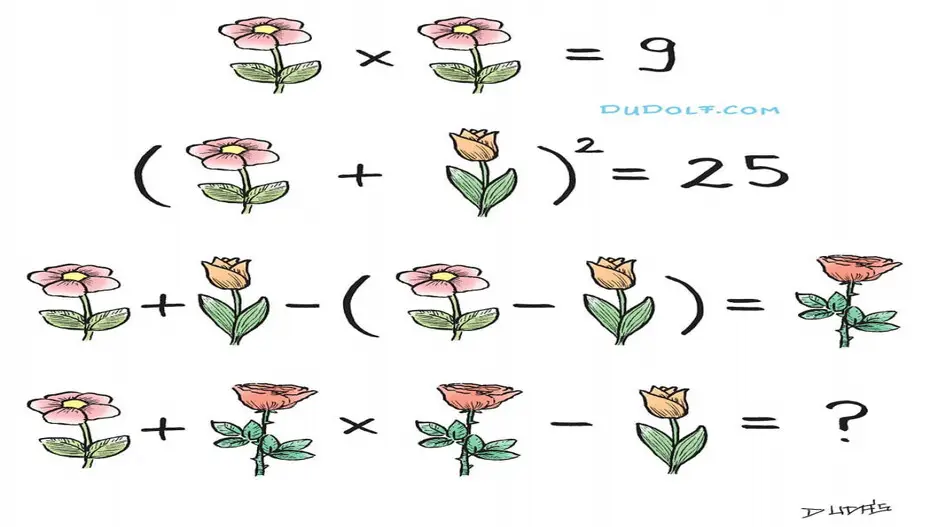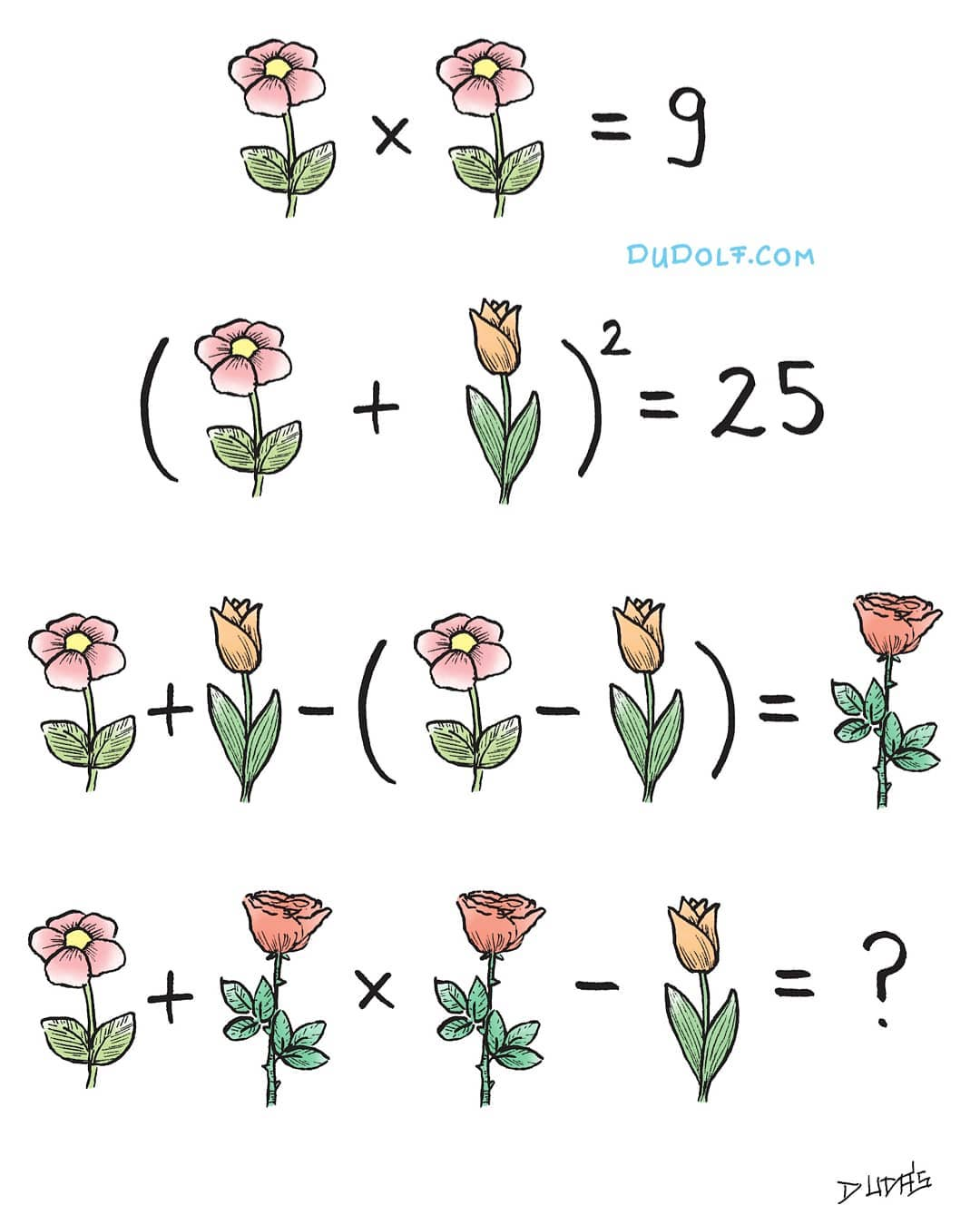•• Home
• News
• Trending
• Only people with a high IQ can solve this math puzzle in 11 seconds

# Only people with a high IQ can solve this math puzzle in 11 seconds

The puzzle was shared by  Hungarian graphic artist Gergely Dudas on his social media pages.Photo: Instagram/thedudolf

Only people with a high IQ can solve this math puzzle in 11 seconds

Math riddles are exciting, engaging and fun to solve. These math puzzles are for all grades and ages. Try to test your mathematical knowledge and visual perception with this math puzzle. Let us solve this math picture puzzle within 11 seconds.

In this Math Puzzle, we have to find the values of flowers to solve this challenging math riddle. Are you a math genius? Only people with a high IQ can solve this math puzzle in 11 seconds.

The puzzle was shared by  Hungarian graphic artist Gergely Dudas on his social media pages.Pic Credit: Instagram/thedudolf

## Let us first summarise the equations here:

1 Pink flower X 1 Pink flower = 9…………… (Row 1)

(1 Pink Flower + 1 Tulip) 2 = 25 ……………. (Row 2)

1 Pink Flower + 1 Tulip – (1 Pink Flower – 1 Tulip) =  1 Rose …………… (Row 3)

1 Pink Flower + 1 Rose X 1 Rose – 1 Tulip =? ………. (Row 4)

So, we need to find the values of Pink, Tulip and Rose flower to solve Row 4.

## Let us begin solving!

In Row 1 equation, we have 1 Donald Duck + 2 Mickey Mouse – 2 Jerry = 5

Now, if we notice the Row 2 equation, we have 2 Mickey Mouse – 2 Jerry = 4

So, we can substitute the information from Row 2 in the Row 1 equation.

We now get,

=> 1 Pink flower X 1 Pink flower = 9

=> 1 Pink flower = 3

Hence, the value of 1 Pink flower = 3

Moving on,

We shall use the value of 1 Pink flower in the Row 2 equation to solve further.

(1 Pink Flower + 1 Tulip) 2 = 25

(3 + 1 Tulip) 2 = 25

1 Tulip flower = 2

Moving on, we shall use the value of 1 Tulip flower in Row 3 to solve further.

In row 3,  we have

1 Pink Flower + 1 Tulip – (1 Pink Flower – 1 Tulip) =  1 Rose

3 + 2 – (3 – 2) = 1 Rose

5 – 1= 1 Rose

4 = 1 Rose

Hence, the value of 1 Rose= 0

Now, we shall solve Row 4 as we have found the values of Pink, Tulip and Rose flowers.

In Row 4, we have 1 Pink Flower + 1 Rose X 1 Rose – 1 Tulip =?

=> 3 + 4 X 4 – 2 =?

=> 3 + 16 – 2 = ?

=> 19 – 2 = 17

Hence, the final answer for this math puzzle is 17.

Hope you have had fun solving this math puzzle.